Disclaimer: This is an example of a student written essay.
Click here for sample essays written by our professional writers.

Any scientific information contained within this essay should not be treated as fact, this content is to be used for educational purposes only and may contain factual inaccuracies or be out of date.

# Determination of the Focal Length of a Convex Lens

Info: 4987 words (20 pages) Essay
Published: 9th Mar 2018

• Shaikh Ilyas

AIM: To determine the focal length of converging lens and it’s radius of curvature.

HYPOTHESIS: The relationship between u and v and the focal length f for a convex lens is given by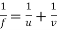. Where f is the focal length, u is the distance between the object and the lens v is the distance between the image and the lens. Real and Virtual Images: Lenses produce images by refraction that are said to be either real or virtual.

• Real images are created by the convergence of rays and can be projected onto a screen; real images form on the side of the lens that is opposite to the object and by convention have a positive image distance value;
• Virtual images are formed by the apparent extrapolation of diverging rays and cannot be formed on a screen, whereas virtual images form on the same side of the lens as the object and have a negative image distance value.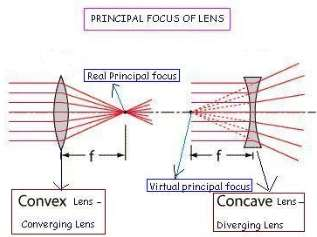BACKGROUND: For a thin double convex lens,refractionacts to focus all parallel rays to a point referred to as the principal focal point. The distance from the lens to that point is the principal focal length f of the lens. Below is the derivation of the lens formula

Following graphic illustrates a simple lens model: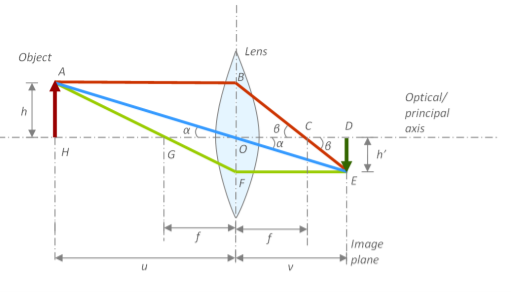where,

h= height of the object

h’= height of the object projected in an image

G and C = focal points

f= focal distance

u= Distance between the object and the focal point

O= Centre of the lens

v= Distance between the centre of the lens and image plane

Assumptions

1. Lens is very thin
2. Optical axis is perpendicular to image plane

Provingis true.

Proof

In ΔAHO,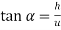In ΔEDO,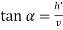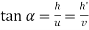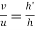—– (1)

In ΔBOC,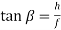In ΔEDC,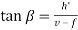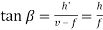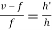—— (2)

Equating equations (1) and (2),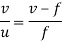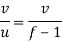Dividing both sides by v,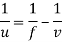Hence the formula is proved.

VARIABLES:

Independent: Distance between the candle and the lens

Dependent: Distance (v) from the image to the lens

Control:

• This experiment was conducted in an almost dark room.
• Same sheet of paper used as the screen.
• A stable candle flame
• The time taken for a sharp and focused image to settle
• The size of the candle.

METHOD FOR CONTROLLING VARIABLES: Made sure that the room was sufficiently dark enough to carry out this experiment as smoothly as possible without any entrance of light from the outside. So I pulled down the blinds of the windows and also made sure that there was no draught present in the room that can make the candle flame unstable. Moreover, I waited for around 6-7 seconds for the image to be seen as sharp and focused. And throughout this experiment I used candles of the same make and size.

APPARATUS REQUIRED:

• 2 meter rules
• A white screen
• Candle
• Convex lens

PROCEDURE:

I divided this experiment in to 2 parts, A and B. In part A, I experimented using a single lens at a time, while in part B, I used 2 lens in contact at a time.

Part A:

1. Firstly I set up the apparatus as shown in Figure 1 above by making the distances v and u the same. So the image observed on a plain white screen was focused and clear
2. Recorded the value of the lengths u and v and thereby marking these original points using a chalk on the bench.
3. Then I adjusted the length of u by moving it away from the lens by 5cm. Consequently, I adjusted the length of v until a sharp and focused image was seen.
4. Recorded this distance of u and v
5. Repeated step 3 – 4 for 7 different values of u by increasing the distance by 5 cm in each step. And recorded the values of u and v for every increment.
6. Then I placed the candle and the screen back in their original marked positions.
7. Finally, repeated the steps 1-8 by using different convex lenses A, B, C, D and E.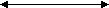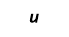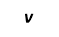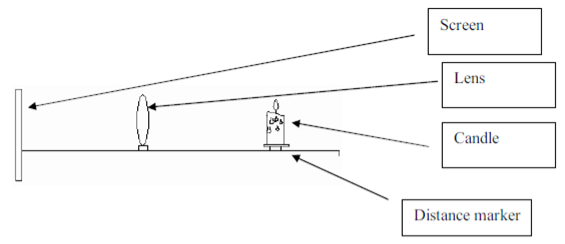Figure 1: Setup of the apparatus for Part A

Part B:

1. Firstly I set up the apparatus as shown in Figure 2 by making the distances v and u the same. So the image observed on a plain white screen was focused and clear
2. Recorded the value of the lengths u and v and thereby marking these original points using a chalk on the bench.
3. Then I adjusted the length of u by moving it away from the lens by 5cm. Consequently, I adjusted the length of v until a sharp and focused image was seen. Recorded this distance of u and v
4. Repeated step 3 – 4 for 4 different values of u by increasing the distance by 5 cm in each step. And recorded the values of u and v for every increment.
5. Repeated the above steps 1-5, thrice.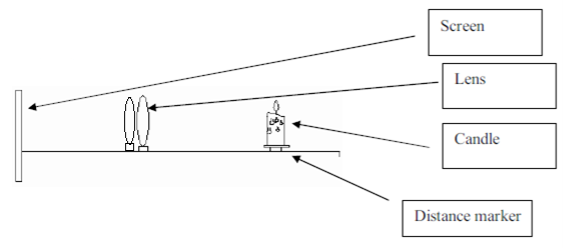Figure 2: Setup of the apparatus for Part B

DATA COLLECTION AND PROCESSING:

• Part A:

Table 1: Data collected for convex lens A

 u (distance between the lens and candle)+ 0.1cm v (distance between the lens and screen)+ 0.1cm 15.0 25.1 20.0 21.5 25.0 17.0 30.0 14.7 35.0 14.2 40.0 13.6 45.0 13.0

Table 2: Data collected for convex lens B

 u (distance between the lens and candle)+ 0.1cm v (distance between the lens and screen)+ 0.1cm 15.0 28.9 20.0 24.2 25.0 19.2 30.0 15.8 35.0 13.9 40.0 13.2 45.0 12.7

Table 3: Data collected for convex lens C

 u (distance between the lens and candle)+ 0.1cm v (distance between the lens and screen)+ 0.1cm 15.0 24.6 20.0 21.1 25.0 16.5 30.0 14.3 35.0 13.9 40.0 13.4 45.0 12.9

Table 4: Data collected for convex lens D

 u (distance between the lens and candle)+ 0.1cm v (distance between the lens and screen)+ 0.1cm 15.0 28.7 20.0 23.6 25.0 17.4 30.0 14.9 35.0 14.0 40.0 13.4 45.0 13.0

Table 5: Data collected for convex lens E

 u (distance between the lens and candle)+ 0.1cm v (distance between the lens and screen)+ 0.1cm 15.0 25.8 20.0 20.1 25.0 15.4 30.0 14.3 35.0 13.9 40.0 13.1 45.0 12.5
• Part B:

Table 6: Data collected for Trial 1

 u (distance between the lens and candle)+ 0.1cm v (distance between the lens and screen)+ 0.1cm 30.0 60 40.0 38 50.0 33 60.0 30.1

Table 7: Data collected for Trial 2

 u (distance between the lens and candle)+ 0.1cm v (distance between the lens and screen)+ 0.1cm 30.0 58.7 40.0 37.8 50.0 32.6 60.0 30

Table 8: Data collected for Trial 3

 u (distance between the lens and candle)+ 0.1cm v (distance between the lens and screen)+ 0.1cm 30.0 61.5 40.0 38.7 50.0 33.2 60.0 29.6

Using the formula, R = 2f I can calculate the value for the radius of curvature. The value of f can be found using the equation.

• Part A:

Table 9:Data processing for convex lens A

 u (distance between the lens and candle) + 0.1cm v (distance between the lens and screen) + 0.1cm Focal length (f) (cm) Radius of curvature (R) (cm) (f-x) (f-x)2 15 25.1 9.39 18.78 -0.62 0.38603 20 21.5 10.36 20.72 0.35 0.12328 25 17.0 10.12 20.24 0.11 0.01182 30 14.7 9.87 19.73 -0.14 0.02090 35 14.2 10.10 20.20 0.09 0.00833 40 13.6 10.15 20.30 0.14 0.01930 45 13.0 10.09 20.17 0.08 0.00576 Mean(f) = 10.01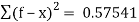Standard deviation: δm =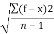=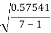= 0.30967

Therefore, the focal length is 10.01+ 0.31 cm

The % error =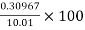= 3.1%

Table 10:Data processing for convex lens B

 u (distance between the lens and candle) + 0.1cm v (distance between the lens and screen) + 0.1cm Focal length (f) (cm) Radius of curvature (R) (cm) (f-x) (f-x)2 15 28.9 9.87 19.75 -0.38 0.14761 20 24.2 10.95 21.90 0.69 0.47792 25 19.2 10.86 21.72 0.60 0.36098 30 15.8 10.35 20.70 0.09 0.00818 35 13.9 9.95 19.90 -0.31 0.09612 40 13.2 9.92 19.85 -0.33 0.11162 45 12.7 9.90 19.81 -0.35 0.12548 Mean(f) = 10.26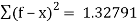Standard deviation: δm === 0.47044

Therefore, the focal length is 10.26+ 0.47 cm

The % error =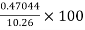= 4.6%

Table 11:Data processing for convex lens C

 u (distance between the lens and candle) + 0.1cm v (distance between the lens and screen) + 0.1cm Focal length (f) (cm) Radius of curvature (R) (cm) (f-x) (f-x)2 15 24.6 9.32 18.64 -0.57 0.32564 20 21.1 10.27 20.54 0.38 0.14350 25 16.5 9.94 19.88 0.05 0.00259 30 14.3 9.68 19.37 -0.20 0.04197 35 13.9 9.95 19.90 0.06 0.00361 40 13.4 10.04 20.07 0.15 0.02209 45 12.9 10.03 20.05 0.14 0.01879 Mean(f) = 9.89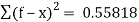Standard deviation: δm ==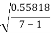= 0.30500

Therefore, the focal length is 9.89+ 0.31 cm

The % error =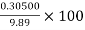= 3.1%

Table 12:Data processing for convex lens D

 u (distance between the lens and candle) + 0.1cm v (distance between the lens and screen) + 0.1cm Focal length (f) (cm) Radius of curvature (R) (cm) (f-x) (f-x)2 15 28.7 9.85 19.70 -0.29 0.08633 20 23.6 10.83 21.65 0.68 0.46324 25 17.4 10.26 20.52 0.11 0.01308 30 14.9 9.96 19.91 -0.19 0.03595 35 14.0 10.00 20.00 -0.15 0.02105 40 13.4 10.04 20.07 -0.11 0.01158 45 13.0 10.09 20.17 -0.06 0.00346 Mean(f) = 10.15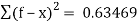Standard deviation: δm ==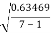= 0.32524

Therefore, the focal length is 10.15+ 0.33 cm

The % error =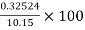= 3.2%

Table 13:Data processing for convex lens E

 u (distance between the lens and candle) + 0.1cm v (distance between the lens and screen) + 0.1cm Focal length (f) (cm) Radius of curvature (R) (cm) (f-x) (f-x)2 15 25.8 9.49 18.97 -0.28 0.07574 20 20.1 10.02 20.05 0.26 0.06992 25 15.4 9.53 19.06 -0.23 0.05327 30 14.3 9.68 19.37 -0.08 0.00586 35 13.9 9.95 19.90 0.19 0.03548 40 13.1 9.87 19.74 0.11 0.01159 45 12.5 9.78 19.57 0.02 0.00049 Mean(f) = 9.76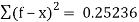Standard deviation: δm ==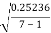= 0.20508

Therefore, the focal length is 9.76 + 0.20508 cm

The % error =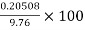= 2.1%

• Part B:

Table 14: Data processing for Trial 1

 u (distance between the lens and candle) + 0.1cm v (distance between the lens and screen) + 0.1cm Focal length (f) (cm) Radius of curvature (R) (cm) (f-x) (f-x)2 30 60.0 20.00 40.00 0.15 0.02168 40 38.0 19.49 38.97 -0.37 0.13366 50 33.0 19.88 39.76 0.03 0.00072 60 30.1 20.04 40.09 0.19 0.03672 Mean(f) = 19.85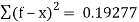Standard deviation: δm === 0.43905

Therefore, the focal length is 19.85 + 0.44cm

The % error =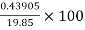= 2.2%

Table 15: Data processing for Trial 2

 u (distance between the lens and candle) + 0.1cm v (distance between the lens and screen) + 0.1cm Focal length (f) (cm) Radius of curvature (R) (cm) (f-x) (f-x)2 30 58.7 19.85 39.71 0.10 0.00961 40 37.8 19.43 38.87 -0.32 0.10300 50 32.6 19.73 39.47 -0.02 0.00047 60 30.0 20.00 40.00 0.24 0.05984 Mean(f) = 19.76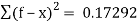Standard deviation: δm === 0.16976

Therefore, the focal length is 19.76 + 0.17 cm

The % error =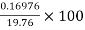= 0.9%

Table 16: Data processing for Trial 3

 u (distance between the lens and candle) + 0.1cm v (distance between the lens and screen) + 0.1cm Focal length (f) (cm) Radius of curvature (R) (cm) (f-x) (f-x)2 30 61.5 20.16 40.33 0.26 0.06875 40 38.7 19.67 39.34 -0.23 0.05387 50 33.2 19.95 39.90 0.05 0.00252 60 29.6 19.82 39.64 -0.08 0.00645 Mean(f) = 19.90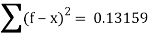Standard deviation: δm === 0.14809

Therefore, the focal length is 19.90 + 0.15 cm

The % error =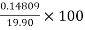= 2.2%

CALCULATIONS AND DATA PRESENTATION:

Table 17: Data presentation for Convex lens A

<

## Cite This Work

To export a reference to this article please select a referencing stye below:

View all

## DMCA / Removal Request

If you are the original writer of this essay and no longer wish to have your work published on UKEssays.com then please:

Related Services

Our academic writing and marking services can help you!

Related Lectures

Study for free with our range of university lectures!Freelance Writing Jobs

Looking for a flexible role?
Do you have a 2:1 degree or higher?

Study Resources

Free resources to assist you with your university studies!

We've received widespread press coverage since 2003We can help with your essayPart of All Answers Ltd

Useful Resources(cm)-1(cm)-1(cm)-1